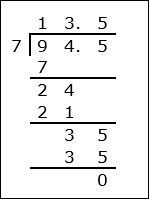# Division of a Decimal by a Whole Number

Division of a decimal by a whole number is similar to whole number division.

Rules for Decimal Division by a Whole Number

• We use division or long division of decimals by whole numbers as if the decimals were whole numbers ignoring the decimal points.

• In the quotient that we get, we place the decimal point in the same spot as in the number that is being divided.

Divide 74.4 ÷ 4

### Solution

Step 1:

74.4 has one decimal place. 4 has no decimal places.

First we divide 744 ÷ 4 ignoring the decimal point.

744 ÷ 4 = 186

Step 2:

Since dividend has one decimal place, the quotient is written with one decimal place to get the answer.

Therefore,

74.4 ÷ 4 = 18.6

Step 3:

Using long division,So 74.4 ÷ 4 = 18.6

Divide 94.5 ÷ 7

### Solution

Step 1:

94.5 has one decimal place. 7 has no decimal places.

First we divide 945 ÷ 7 ignoring the decimal point.

945 ÷ 7 = 135

Step 2:

Since dividend has one decimal place, the quotient is written with one decimal place to get the answer.

Therefore,

94.5 ÷ 7 = 13.5

Step 3:

Using long division,So 94.5 ÷ 7 = 13.5